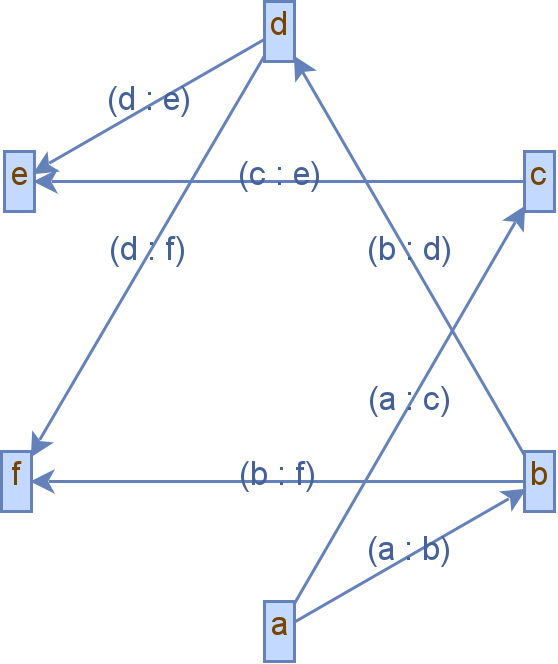# Java Graph Libraries

A graph is a data structure in Java consisting of nodes and their edges. A node represents the data, while the edges tell the relationship between the nodes.

In the sections below, we will check out two libraries that help us implement the concept of a graph in Java.

## Use the `JGraphtT` Library to Create a Graph in Java

`JGraphT` is a third-party library containing classes and methods to implement different graphs like a directed graph, weighted graph, pseudograph, etc. We can perform various operations like traversal using graph algorithms.

In the program, we create an object of the `DefaultDirectedGraph` class, a graph structure class in `JGraphT`, and return an instance of `Graph`. We pass an edge class `DefaultEdge` in the constructor of the `DefaultDirectedGraph` class.

The `Graph` takes two type parameters for the node type and the edge type, where we use `String` as the type of data in the node while the edge should be `DefaultEdge`.

We add the vertices or nodes to the `graph` using the `addVertex()` method. Next, we need to specify the edges between the nodes using the `addEdge()` function.

We pass two arguments in the `addEdges()` function: the edge’s source and target. The first edge goes from the node with data `a` to the node with `b`.

We have a graph with both vertices and edges. Still, we can also use the `JGraphT` library to visualize the graph using the `JGraphX` library, which can be imported into the project using the following maven dependency.

``````<dependency>
<groupId>com.github.vlsi.mxgraph</groupId>
<artifactId>jgraphx</artifactId>
<version>4.2.2</version>
</dependency>
``````

After we import the `JGraphX` library, we create an object of `JGraphXAdapter` and pass the `graph` object in its constructor that returns the `jGraphXAdapter` reference. Now we need to set the layout to display the nodes and edges.

It is done using the `mxCircleLayout` object that extends `mxGraphLayout`, and we pass the `jGraphXAdapter` to the constructor of `mxCircleLayout` class that returns a reference of type `mxGraphLayout`.

We execute the layout using the `mxGraphLayout.execute()` that takes the parent of the layout as an argument.

Now we call `createBufferedImage()` of the `mxCellRenderer` class that takes six arguments: the `JGraphXAdapter` object, the number of cells, the scale or size of the image, the background of the generated image, to enable or disable anti-aliasing, and to clip the image.

We pass all the respective arguments and get a `BufferedImage` object.

Finally, we create a file where the generated file will be stored using the `File()` object and pass the file name and its path in it on writing the image; we use `ImageIO.write()` that takes the `BufferedImage` object, the format of the image to be written and the `File` object.

``````import com.mxgraph.layout.*;
import com.mxgraph.util.*;
import org.jgrapht.Graph;
import org.jgrapht.graph.DefaultDirectedGraph;
import org.jgrapht.graph.DefaultEdge;

import javax.imageio.ImageIO;
import java.awt.*;
import java.awt.image.BufferedImage;
import java.io.File;
import java.io.IOException;

public class JavaExample {
public static void main(String[] args) throws IOException {

Graph<String, DefaultEdge> graph = new DefaultDirectedGraph<>(DefaultEdge.class);

BufferedImage bufferedImage = mxCellRenderer.createBufferedImage(jGraphXAdapter, null, 3, Color.WHITE, true, null);

File newFIle = new File("graph.png");
ImageIO.write(bufferedImage, "PNG", newFIle);

}
}
``````

Output:## Use the Guava Library to Create a Graph in Java

Another library to implement a graph in Java is the Guava library that we can use in our project using the following maven dependency.

``````<dependency>
<artifactId>guava</artifactId>
<version>31.0.1-jre</version>
</dependency>
``````

Once the dependency is set, we can move to the program.

We use the `GraphBuilder` to call its static method `directed()`, which is used to build directed graphs, and call the `build()` method that returns an empty `MutableGraph` object. We set the type of the node data as String.

We add the node data using the `addNode` method and the edges using the `putEdge()` method. In the `putEdge()` function, we pass the source vertex and the target vertex.

After adding the nodes and their edges, we create a traversable graph by calling the `forGraph()` method of the `Traverser` class and passing the `mutableGraph` object.

We can perform different types of traversal algorithms, but for this example, we traverse the graph using the breadth-first approach by calling the `breadthFirst()` function and pass the node data from where to start the traversal.

The `breadthFirst()` returns an `Iterable` instance that can iterate using a loop and print the nodes.

``````import com.google.common.graph.GraphBuilder;

public class JavaExample {
public static void main(String[] args) {

MutableGraph<String> mutableGraph = GraphBuilder.directed().build();

mutableGraph.putEdge("root", "l1");
mutableGraph.putEdge("root", "r1");
mutableGraph.putEdge("l1", "l2");
mutableGraph.putEdge("l1", "r2");
mutableGraph.putEdge("r1", "l3");
mutableGraph.putEdge("r1", "r3");
mutableGraph.putEdge("l3", "l4");
mutableGraph.putEdge("l3", "r4");

Traverser<String> traversedGraph = Traverser.forGraph(mutableGraph);

for (String s : getDepthFirstResult) {
System.out.println(s);
}

}
}
``````

Output:

``````root
l1
r1
r2
l2
r3
l3
l4
r4
``````